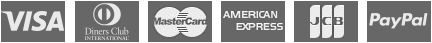DEV

## TMS Analytics & Physics Pack

Delphi library that provides an easy way to evaluate mathematical expressions, calculate symbolic derivatives & integration, solving non linear equations, approximation function calculation and more...

Version history

### Feature overview

TMS Analytics & Physics Pack is a Delphi library for developers that contains special classes to work with analytical expressions in Delphi programs - parse expressions, calculate expression values with multiple parameters, expression based derivatives & integration calculation and so on. In addition, it offers approximation function calculation, solving differential and non-linear equations. TMS Analytics & Physics Pack allows using various physics concepts (such as physical quantities, units of measurement, ...) in Delphi programs.

### Benefits

• 100% Delphi code
• Strongly structured class hierarchy
• Universal algorithms for working with formulae of any complexity and for working with physics concepts
• Many predefined functions and physical entities (physical quantities, units of measurement)
• Easy to introduce new functions for any argument types and new physics concepts (physical quantities, units of measurement and so on)
• Easy to overload operators for any argument types
• Working with Complex numbers, 3D vectors and tensors
• Working with indexed data (arrays, matrixes and higher dimensioned data)
• Analytical (expression based) derivative calculation
• Conditional 'if' function (supporting real, complex and fractions), including derivative evaluation
• Special extension: allows calculation of special functions (Bessel, Legendre) and their derivatives
• Platform independent: can be used in VCL and FMX applications for Windows, macOS, iOS, Android
• Fractions extension: allows using common fractions in math expressions, including making operations like 1/2+1/3 = 5/6, converting floating values to common fractions and vice versa
• Approximation tool: least squares approximation of multidimensional data with arbitrary, user defined basis functions
• Numerical integration: calculating definite integral values for one- and two- dimensional functions
• ODE tool: solving initial value problems for the systems of ordinary differential equations
• Function analysis tool: finding roots and extremums for univariate functions
• Nonlinear tool: solving systems of nonlinear equations

### Help

DEV

#### TMS Analytics & Physics Pack

for Delphi XE5, XE6, XE7, XE8, 10 Seattle, 10.1 Berlin, 10.2 Tokyo (Prof/Enterprise/Architect)

View licensing FAQ
• Includes full source code
• Free updates for a full version cycle (from v3.0 to v3.9) or maximum 2 years of free updates
• Free priority support through email and forum

license for 1 developer € 95